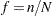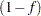# Survey Design Specification

Survey sampling is the process of selecting a probability-based sample from a finite population according to a sample design. You then collect data from these selected units and use them to estimate characteristics of the entire population.

A sample design encompasses the rules and operations by which you select sampling units from the population and the computation of sample statistics, which are estimates of the population values of interest. The objective of your survey often determines appropriate sample designs and valid data collection methodology. A complex sample design can include stratification, clustering, multiple stages of selection, and unequal weighting. The survey procedures can be used for single-stage designs or for multistage designs, with or without stratification, and with or without unequal weighting.

To analyze your survey data with the SURVEYMEANS, SURVEYFREQ, SURVEYREG, SURVEYLOGISTIC, and SURVEYPHREG procedures, you need to specify sample design information for the procedures. This information can include design strata, clusters, and sampling weights. All the survey analysis procedures use the same syntax for specifying sample design information. You provide sample design information with the STRATA, CLUSTER, and WEIGHT statements, and with the RATE= or TOTAL= option in the PROC statement.

If you provide replicate weights for BRR or jackknife variance estimation, you do not need to specify a STRATA or CLUSTER statement. Otherwise, you should specify STRATA and CLUSTER statements whenever your design includes stratification and clustering.

When there are clusters (PSUs) in the sample design, the procedures estimate variance by using the PSUs, as described in the section Variance Estimation. For a multistage sample design, the procedures use only the first stage of the sample design for variance estimation. Therefore, the required input includes only first-stage cluster (PSU) and first-stage stratum identification. You do not need to input design information about any additional stages of sampling.

The following sections provide brief descriptions of basic sample design concepts and terminology used in the survey procedures. See Lohr (2010), Kalton (1983), Cochran (1977), and Kish (1965) for more detailed information.

### Population

Population refers to the target population, which is the group of units (individuals or elements) of interest for study. Often, the primary objective is to estimate certain characteristics of this population, which are called population values. A sampling unit is an individual or element in the target population. A sample is a subset of the population that is selected for the study.

Before you use the survey procedures, you should have a well-defined target population, sampling units, and an appropriate sample design.

In order to select a sample according to your sample design, you need to have a list of sampling units in the population. This is called a sampling frame. PROC SURVEYSELECT uses probability-based selection methods to select a sample from a sampling frame.

### Stratification

Stratified sampling involves selecting samples independently within strata, which are nonoverlapping subgroups of the survey population. Stratification controls the distribution of the sample size in the strata. It is widely used to meet a variety of survey objectives. For example, with stratification you can ensure adequate sample sizes for subgroups of interest, including small subgroups, or you can use stratification to improve the precision of overall estimates. To improve precision, units within strata should be as homogeneous as possible for the characteristics of interest.

### Clustering

Cluster sampling involves selecting clusters, which are groups of sampling units. For example, clusters might be schools, hospitals, or geographical areas, and sampling units might be students, patients, or citizens. Cluster sampling can provide efficiency in frame construction and other survey operations. However, it can also result in a loss in precision of your estimates, compared to a nonclustered sample of the same size. To minimize this effect, units within clusters should be as heterogeneous as possible for the characteristics of interest.

### Multistage Sampling

In multistage sampling, you select an initial (first-stage) sample that is based on groups of elements in the population, which are called primary sampling units (PSUs).

Then you create a second-stage sample by drawing a subsample from each selected PSU in the first-stage sample. By repeating this operation, you can select a higher-stage sample. If you include all the elements from the selected primary sampling units, then the two-stage sample is a cluster sample.

### Sampling Weights

Sampling weights, which are also known as survey weights, are positive values associated with the units in your sample. Ideally, the weight of a sampling unit should be the "frequency" that the sampling unit represents in the target population.

Often, sampling weights are the reciprocals of the selection probabilities for the sampling units. When you use PROC SURVEYSELECT, the procedure generates the sampling weight component for each stage of the design, and you can multiply these sampling weight components to obtain the final sampling weights. Sometimes, sampling weights also include nonresponse adjustments, postsampling stratification, or regression adjustments by using supplemental information.

When the sampling units have unequal weights, you must provide the weights to the survey analysis procedures. If you do not specify sampling weights, the procedures use equal weights in the analyses.

### Population Totals and Sampling Rates

If you use Taylor series variance estimation, the survey procedures include a finite population correction factor in the analysis if you input either the sampling rate or the population total.

The sampling rate is the ratio of the sample size (the number of sampling units in the sample)to the population size (the total number of sampling units in the target population),. This ratio is also called the sampling fraction. If you select a sample without replacement, the extra efficiency compared to selecting a sample with replacement can be measured by the finite population correction (fpc) factor,.

To include a finite population correction factor in your analysis, you can input either the sampling rate or the population total. Otherwise, the procedures do not use the fpc in computing variance estimates. For fairly small sampling fractions, it is appropriate to ignore this correction. See Cochran (1977) and Kish (1965) for details.

As discussed in the section Variance Estimation, for a multistage sample design, the procedures use only the first stage of the sample design for variance estimation. Therefore, if you are specifying the sampling rate, you should input the first-stage sampling rate, which is the ratio of the number of PSUs in the sample to the total number of PSUs in the target population.

If you use BRR or jackknife variance estimate, the procedures do not include a finite population correction in the analysis, and you do not need to input the sampling rate or the population total.